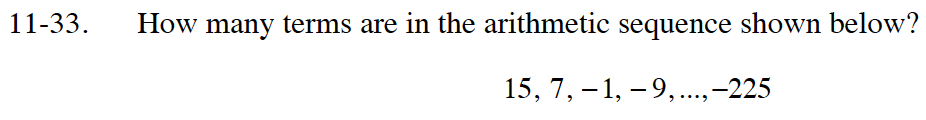### Home > INT1 > Chapter Ch11 > Lesson 11.1.3 > Problem11-33

11-33.
1. How many terms are in the arithmetic sequence shown below? Homework Help ✎

15, 7, −1, −9, ..., −225Write an equation for the arithmetic sequence using anm(n − 1) + a1

Let an = −225 and solve for n.